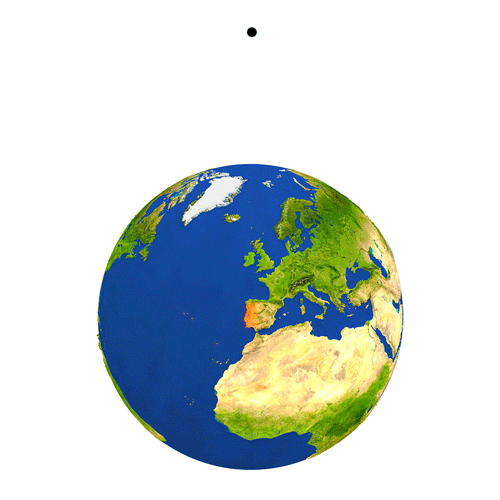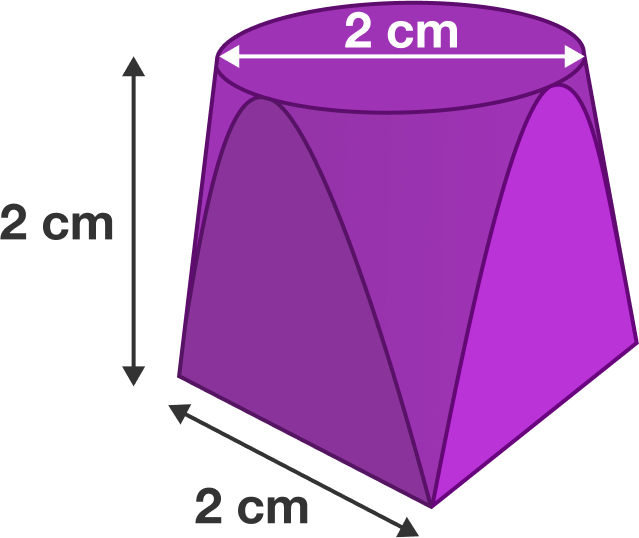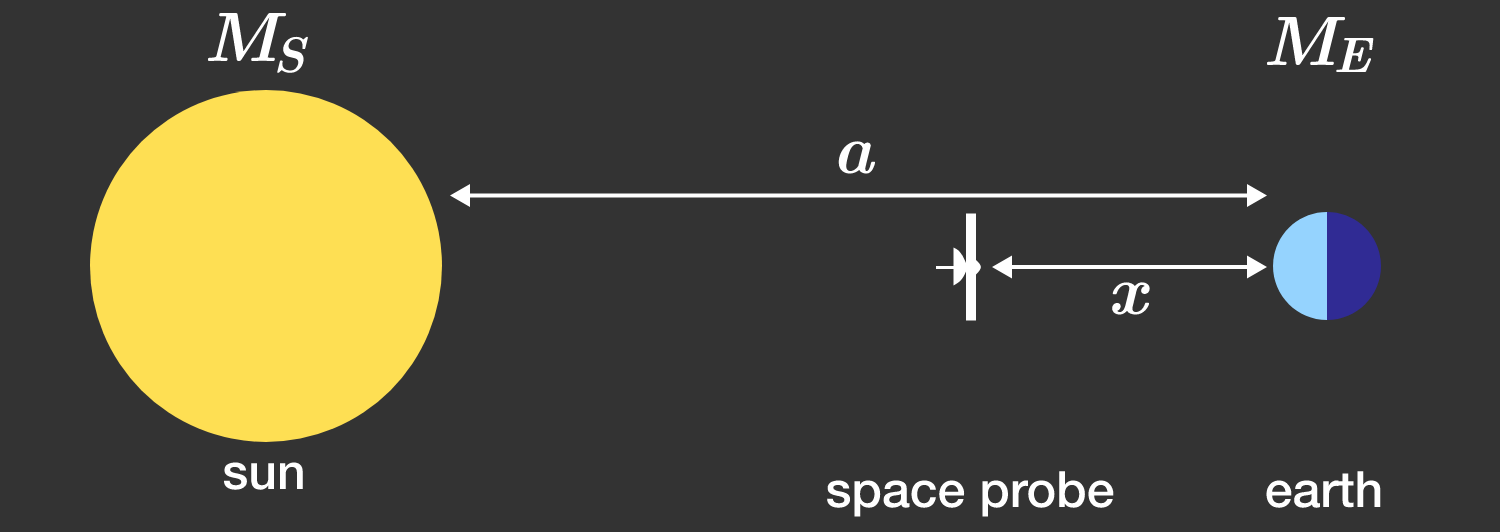# Problems of the Week

Contribute a problem

$S=1^{33} + 2^{33} + 3^{33} + 4^{33} + \cdots + 89^{33}$

What is the units digit of $S?$Assume that Earth is a perfect sphere with radius $R.$ The time $T$ an object takes to fall to the ground from rest from a height of $R$ above the ground is given by $T=\sqrt{\frac Rg}\left(A+\frac {\pi^B}C\right),$ where $A,B,C$ are positive integers.

Find $A+B+C.$

Note: Consider only gravity, and forget about atmospheric effects, air resistance, etc.

After studying various 3D shapes and finding formulas for their volumes, I challenged my students to invent a new shape. Lindsay created a shape $($with height $2 \text{ cm})$ that is circular at the top $($with radius $1\text{ cm})$ but square at the bottom $($with side length $2\text{ cm}).$ Lindsay created this shape from a paraboloid: sliced four times parallel to the paraboloid's axis and two times perpendicular to the paraboloid's axis. Lindsay's shape is pictured below.

Find the volume of Lindsay's shape in $\text{cm}^{3},$ which can be written as $\frac{A}{B}+C\pi$ with $A,B,C$ integers, $A$ and $B$ coprime, and $B$ positive.

Give the value of $A+B+C.$Four nails are randomly fixed on a circular soft board.

Emma takes a red elastic rubber band, stretches it around the nails, and lets go.

What is the probability that the rubber band does not take the shape of a quadrilateral?

Source: Putnam 2006 A-6

There is a special point between Sun and Earth where a spacecraft can be parked, so that it always remains directly between them, at a fixed distance $x$ from Earth, as Earth rotates around the Sun. A spacecraft can remain at this point without using any thrust.

What is the distance $x$ of this point from the Earth in gigameters? $\big(1\text{ gigameter}=10^6\text{ km}\big)$Details and Assumptions:

• The masses of the Earth and the Sun have a ratio of $1: 333,000.$
• The distance between the Earth and the Sun is $a \approx 150 \cdot 10^6 \text{ km}.$
• The radii of the Earth and the Sun are negligibly small.
×